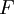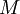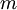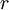# Law of Universal Gravitation•is the gravitational force
•is Newton's gravitational constant
•is the mass of the first mass
•is the mass of the second mass
•is the distance between the two masses